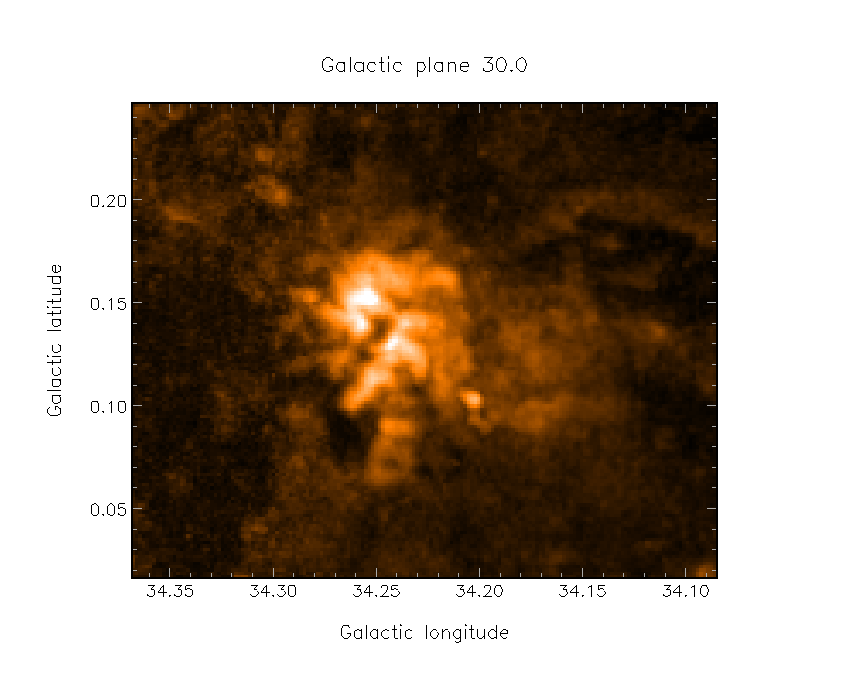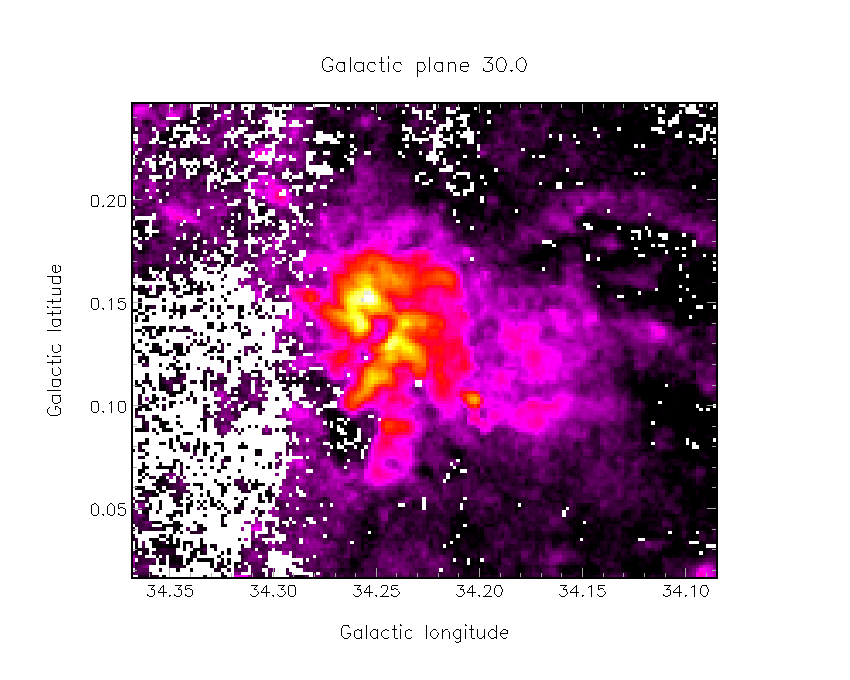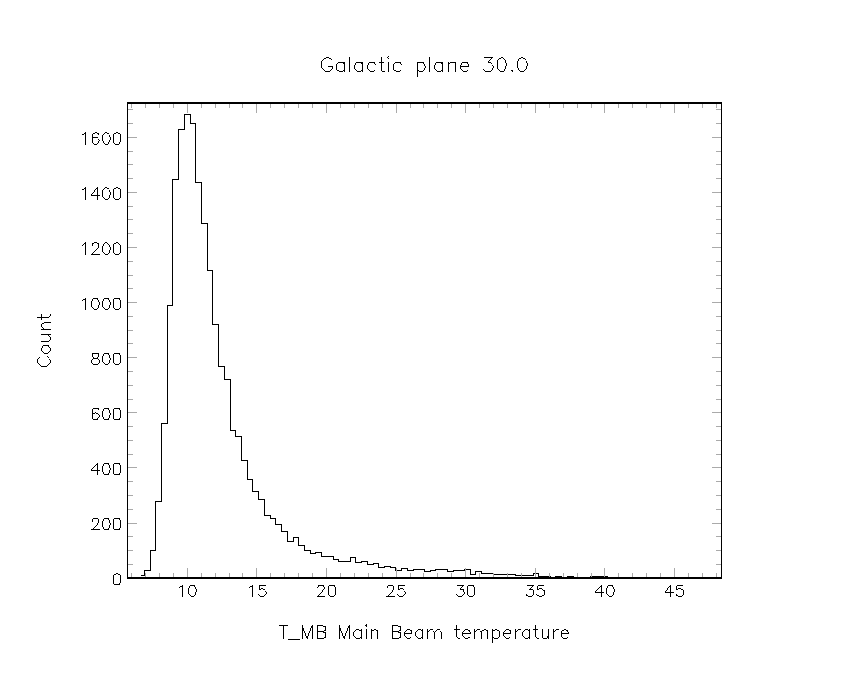# Calculating Excitation Temperatures from 12CO cube¶

Ignoring self absorption and assuming infinite optical depth and Local Thermodynamic Equilibrium, it is possible to estimate the excitation temperature of a line from:

$$T_{B} =\frac{h\nu}{k_B} \left( \frac{1}{\exp[h\nu/k_{B}T_{ex}]} - \frac{1}{\exp[h\nu/k_{B}T_{CMB}]}\right)$$

Where $T_{B}$ is the RJ brightness temperature of the line, $\nu$ is the frequency of the line, $T_{CMB}$ is the CMB temperature, and $T_{ex}$ is the excitation temperature of the line.

We can use the peak temperature measured in our cube as the RJ brightness temperature $T_{B}$. For $^{12}$CO J=3$\rightarrow$2 as observed with HARP, we can then simplify and rearrange this formula to:

$$T_{ex} = \frac{16.59\,K}{ln\left(1 + \frac{16.59\,K}{T_{peak} + 0.036\,K}\right)}$$

To calculate this, we need a 12CO cube, which we will then get the peak temperature of each spectra in it. We then need to apply the above formula to this. This tutorial will take you through the steps of doing this within Starlink.

First of all, we must set up Starlink, change into our working directory, and initialise the KAPPA package of commands. We will also tell Starlink not to prompt us for user input, as we are working inside a jupyter notebook. (You should replace the /star with the correct location of your Starlink installation directory.)

In :
export STARLINK_DIR=/star
source $STARLINK_DIR/etc/profile export ADAM_NOPROMPT=1 mkdir CubeAnalysis cd CubeAnalysis kappa   KAPPA commands are now available -- (Version 2.5-8) Type kaphelp for help on KAPPA commands. Type 'showme sun95' to browse the hypertext documentation. See the 'Release Notes' section of SUN/95 for details of the changes made for this release.  We can then collapse our 12CO cube along the velocity axis. We want to end up with a 2-D cube where the pixel values are the max pixel value found in that spectra. We can use the command collapse with the estimator=max option. In : collapse in=../Data/g34-3-12co_small_trim.sdf axis=VRAD estimator=max out=g34-3-12co_peakcoll.sdf WLIM=0.0   Collapsing pixel axis 3 from pixel -65 to pixel 121 inclusive...  Lets take a quick look at what this map looks like, using KAPPA's display command, and setting some defaults appropriate for PNG output. In : lutwarm dev=peak.png/PNG palentry 0 White device=PNG palentry 1 Black device=PNG echo 'border=1' > style.dat echo 'color(border) = black' >>style.dat echo 'color(numlab) = black' >>style.dat echo 'color(ticks) = grey' >>style.dat display in=g34-3-12co_peakcoll.sdf style=^style.dat dev=peak.png/PNG mode=faint  Data will be scaled from 1.20042586326599 to 22.0331649780273.Before we calculate our excitation temperature, lets check what temperature scale our data is in. We can check the label attribute of the NDF, as shown by ndftrace. In : ndftrace g34-3-12co_peakcoll.sdf   NDF structure /export/data/sgraves/Tutorials/AnalysisHowTos/CubeAnalysis/g34-3-12co_peakcoll: Title: Galactic plane 30.0 Label: TA* corrected antenna temperature Units: K Shape: No. of dimensions: 2 Dimension size(s): 171 x 139 Pixel bounds : -2625:-2455, 11:149 Total pixels : 23769 Data Component: Type : _REAL Storage form: SIMPLE Bad pixels may be present Variance Component: Type : _REAL Storage form: SIMPLE Bad pixels may be present World Co-ordinate Systems: Number of co-ordinate Frames: 5 Current co-ordinate Frame (Frame 5): Frame title : "IAU (1958) galactic coordinates; gnom..." Domain : SKY First pixel centre : 34.3675, 0.0170 Axis 1: Label : Galactic longitude Units : degrees Nominal Pixel scale: 5.96741 arc-sec Axis 2: Label : Galactic latitude Units : degrees Nominal Pixel scale: 5.98365 arc-sec Extensions: FITS <_CHAR*80> History Component: Created : 2012 Oct 13 16:06:57 No. records: 29 Last update: 2019 Nov 01 02:32:36 (COLLAPSE (KAPPA 2.5-8)) Update mode: NORMAL  You can see it is$T^*_A$temperature scale, in units of$K$. We now need to apply a standard calibration to go from the$T^*_A$(corrected antenna temperature) scale to a telescope-independent scale. We will use$\eta_{MB}$, which allows us to convert to main beam temperature, which assumes a source that fills the beam. For HARP, we use the standard value of$\eta_{MB}=0.67\$. We need to divide by the efficiency (cdiv) and set the new temperature scale into the label attribute of the NDF.

In :
cdiv in=g34-3-12co_peakcoll.sdf scalar=0.67 out=12co_peakcoll_tmb.sdf
setlabel ndf=12co_peakcoll_tmb.sdf label='"T_MB Main Beam temperature"'


You can check that this has updated the label by rerunning ndftrace:

In :
ndftrace 12co_peakcoll_tmb.sdf |grep Label

      Label:  T_MB Main Beam temperature
Label              : Galactic longitude
Label              : Galactic latitude


In practice, we probably don't want to include points with a poor detection. We can use errclip to set as BAD all pixels without a SNR of 5.

In :
errclip in=12co_peakcoll_tmb.sdf out=12co_peakcoll_tmb_clip limit=5.0 mode=SNR

  Applying a lower limit on signal-to-noise ratio.
3846 pixels had signal-to-noise ratios less than 5 in
"/export/data/sgraves/Tutorials/AnalysisHowTos/CubeAnalysis/12co_peakcoll_tmb".



We can now calculate our excitation temperature in each pixel. The maths command lets us apply an arbitrary mathematical formula to our map. To apply the formula above, we use:

In :
maths exp="pa/(log(1+pa/(ia+pb)))" pa=16.59 pb=0.036 ia=12co_peakcoll_tmb_clip.sdf out=12co_excitation_temp.sdf


The exp (expression) argument defines the mathemtaical function (using fortran style syntax). The parameters pa and pb refer to constants, and ia refers to an input NDF. These are then given as separate argument to the command. This is a very powerful command, that can take up to 26 separate constant parameters and up to 26 (ia, ib ... iz) input NDFs.

We can now display the excitation temperature map we have made:

In :
palentry 0 White dev=PNG
palentry 1 Black dev=PNG
lutheat dev=PNG
display in=12co_excitation_temp.sdf style=^style.dat device=Tex.png/PNG mode=faint

Data will be scaled from 7.92171716690063 to 44.0677604675293.We can then calculate the histogram of these data points using the histogram command, with 100 bins and using the same style.dat file as we used before. This command will create a plot of the histogram, and also print information to screen about the bins and the number of pixels in each one.

In :
histogram 12co_excitation_temp 100  style=^style.dat accept dev=Tex_histogram.png/PNG

Minimum value is 6.5047860145569 and the maximum is 47.573101043701
Data limits are from 6.50478601455688 to 47.5731010437012.

Histogram for the NDF structure
/export/data/sgraves/Tutorials/AnalysisHowTos/CubeAnalysis/12co_excitation_temp

Title                     : Galactic plane 30.0
NDF array analysed        : Data

6.504786     to   6.915469            9 pixels
6.915469     to   7.326152           26 pixels
7.326152     to   7.736835          101 pixels
7.736835     to   8.147518          280 pixels
8.147518     to   8.558202          562 pixels
8.558202     to   8.968884          988 pixels
8.968884     to   9.379568         1446 pixels
9.379568     to   9.790251         1629 pixels
9.790251     to   10.20093         1683 pixels
10.20093     to   10.61162         1650 pixels
10.61162     to   11.02230         1435 pixels
11.02230     to   11.43298         1286 pixels
11.43298     to   11.84367         1118 pixels
11.84367     to   12.25435          921 pixels
12.25435     to   12.66503          767 pixels
12.66503     to   13.07572          722 pixels
13.07572     to   13.48640          537 pixels
13.48640     to   13.89708          513 pixels
13.89708     to   14.30777          427 pixels
14.30777     to   14.71845          357 pixels
14.71845     to   15.12913          316 pixels
15.12913     to   15.53981          285 pixels
15.53981     to   15.95050          228 pixels
15.95050     to   16.36118          216 pixels
16.36118     to   16.77186          196 pixels
16.77186     to   17.18255          168 pixels
17.18255     to   17.59323          134 pixels
17.59323     to   18.00391          148 pixels
18.00391     to   18.41460          119 pixels
18.41460     to   18.82528          100 pixels
18.82528     to   19.23596           90 pixels
19.23596     to   19.64665           94 pixels
19.64665     to   20.05733           79 pixels
20.05733     to   20.46801           79 pixels
20.46801     to   20.87869           67 pixels
20.87869     to   21.28938           60 pixels
21.28938     to   21.70006           59 pixels
21.70006     to   22.11074           76 pixels
22.11074     to   22.52143           55 pixels
22.52143     to   22.93211           60 pixels
22.93211     to   23.34279           48 pixels
23.34279     to   23.75348           52 pixels
23.75348     to   24.16416           39 pixels
24.16416     to   24.57484           42 pixels
24.57484     to   24.98553           39 pixels
24.98553     to   25.39621           26 pixels
25.39621     to   25.80689           34 pixels
25.80689     to   26.21758           28 pixels
26.21758     to   26.62826           30 pixels
26.62826     to   27.03894           30 pixels
27.03894     to   27.44963           25 pixels
27.44963     to   27.86031           27 pixels
27.86031     to   28.27099           32 pixels
28.27099     to   28.68167           33 pixels
28.68167     to   29.09236           24 pixels
29.09236     to   29.50304           27 pixels
29.50304     to   29.91372           27 pixels
29.91372     to   30.32441           30 pixels
30.32441     to   30.73509           14 pixels
30.73509     to   31.14577           24 pixels
31.14577     to   31.55646           17 pixels
31.55646     to   31.96714           18 pixels
31.96714     to   32.37782           14 pixels
32.37782     to   32.78851           12 pixels
32.78851     to   33.19919           13 pixels
33.19919     to   33.60987           14 pixels
33.60987     to   34.02056           10 pixels
34.02056     to   34.43124           11 pixels
34.43124     to   34.84192           10 pixels
34.84192     to   35.25261           16 pixels
35.25261     to   35.66329            7 pixels
35.66329     to   36.07397            5 pixels
36.07397     to   36.48465            4 pixels
36.48465     to   36.89534            6 pixels
36.89534     to   37.30602            2 pixels
37.30602     to   37.71671            5 pixels
37.71671     to   38.12739            2 pixels
38.12739     to   38.53807            4 pixels
38.53807     to   38.94875            2 pixels
38.94875     to   39.35944            5 pixels
39.35944     to   39.77012            6 pixels
39.77012     to   40.18081            5 pixels
40.18081     to   40.59149            2 pixels
40.59149     to   41.00217            4 pixels
41.00217     to   41.41285            1 pixels
41.41285     to   41.82354            2 pixels
41.82354     to   42.23422            0 pixels
42.23422     to   42.64490            0 pixels
42.64490     to   43.05558            1 pixels
43.05558     to   43.46627            1 pixels
43.46627     to   43.87695            1 pixels
43.87695     to   44.28764            0 pixels
44.28764     to   44.69832            3 pixels
44.69832     to   45.10900            0 pixels
45.10900     to   45.51968            0 pixels
45.51968     to   45.93037            0 pixels
45.93037     to   46.34105            1 pixels
46.34105     to   46.75173            0 pixels
46.75173     to   47.16242            0 pixels
47.16242     to   47.57310            2 pixels